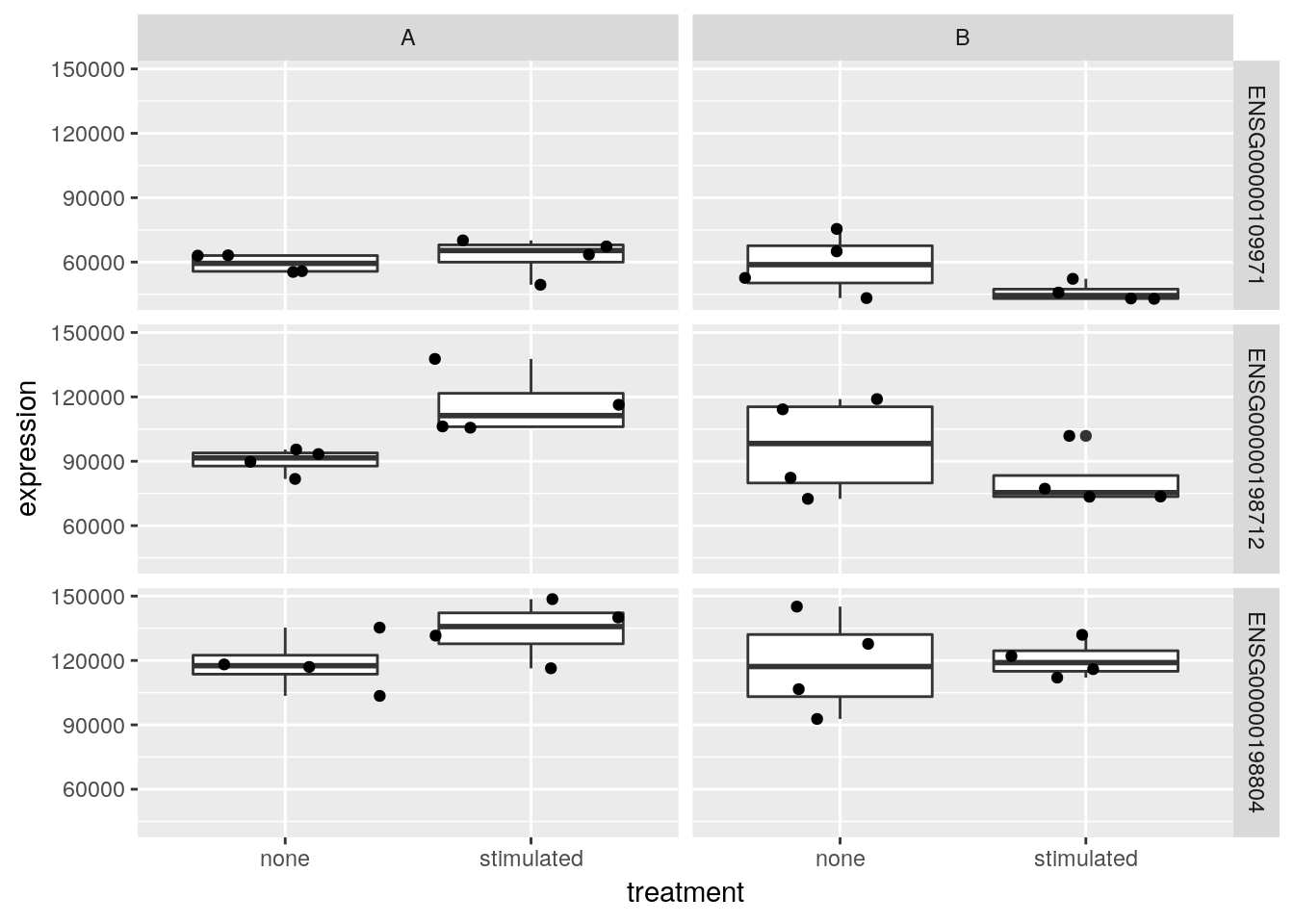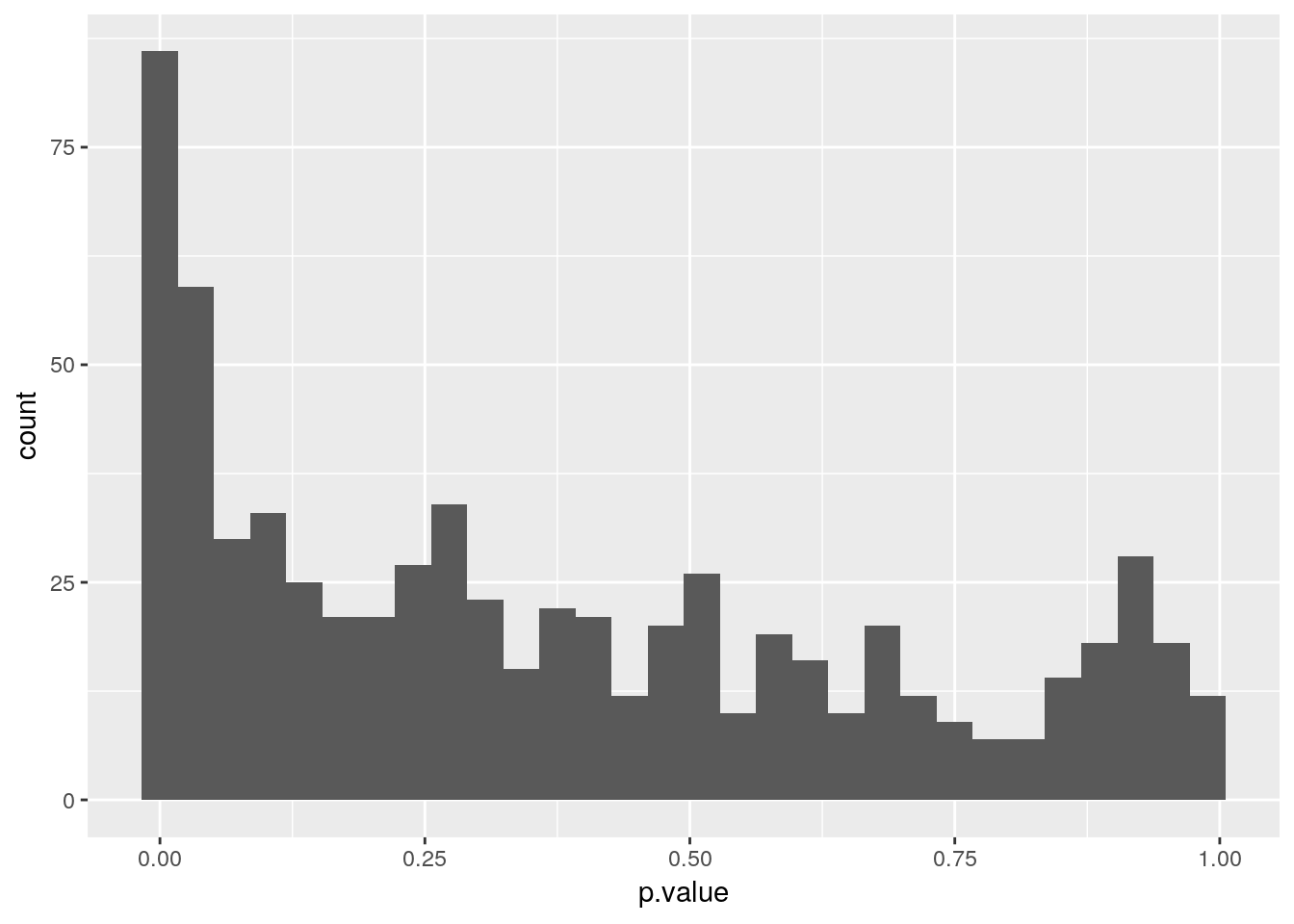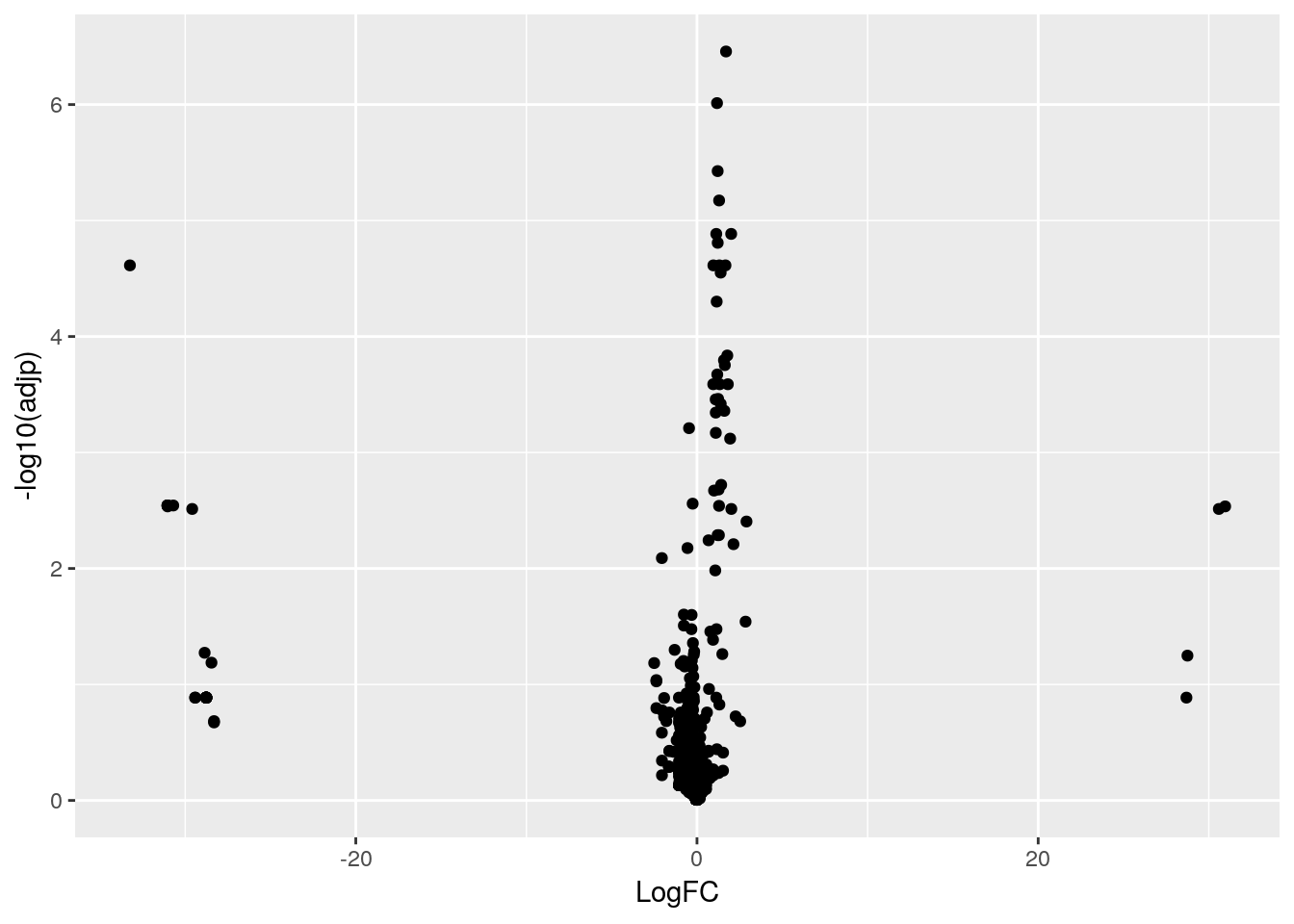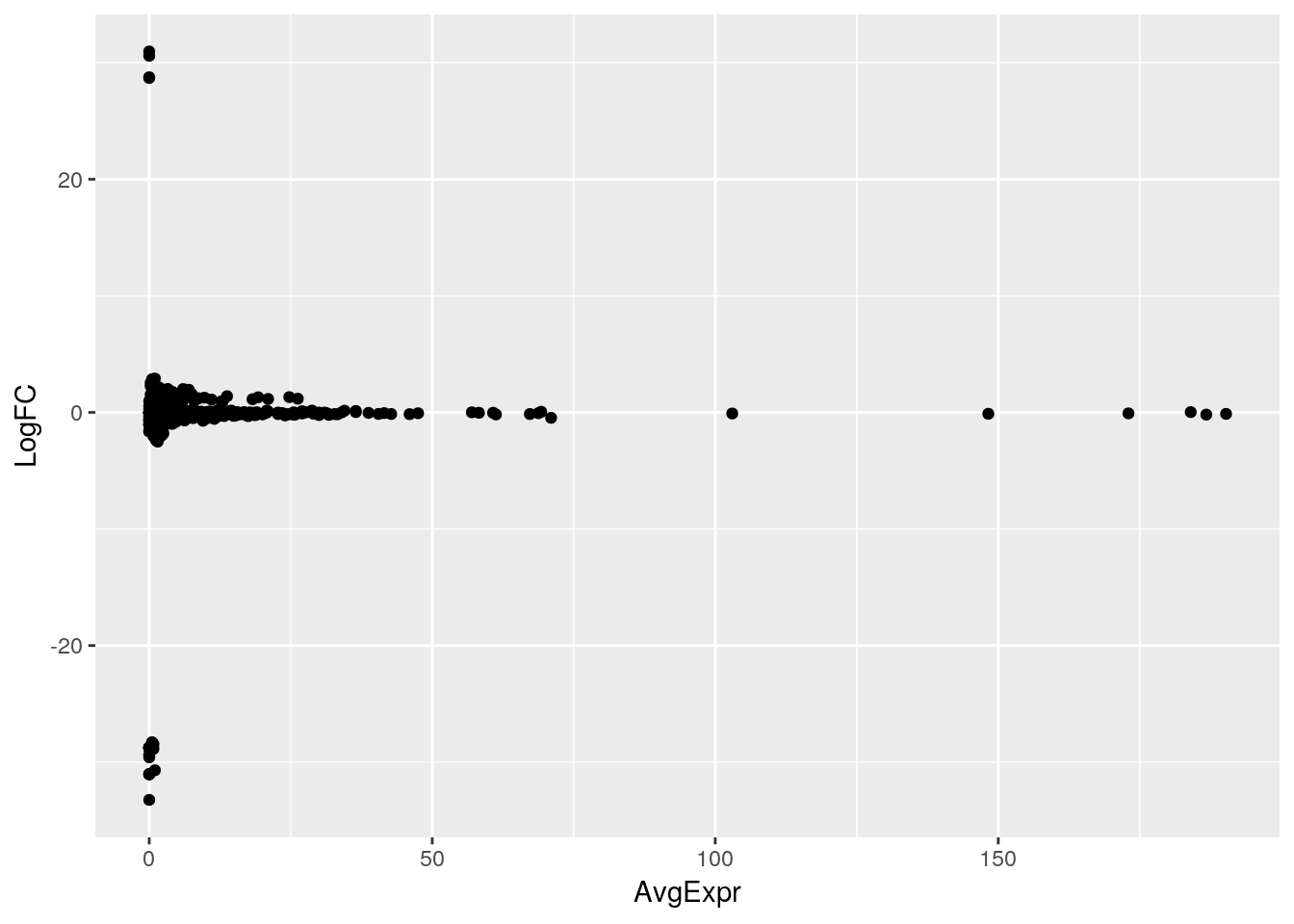# Data Carpentry - additional R exercises

## RNASeq data and annotation

1. Import the data from two tab-separated files into R. The kem_counts.tsv file contains RNA-Seq expression counts for 13 genes and 18 samples and kem_annot.tsv contains annotation about each sample. Read the data into two tibbles named kem and annot respectively and familiarise yourself with the content of the two new tables.

2. Convert the counts data into a long table format and annotation each sample using the experimental design.

3. Identity the three transcript identifiers that have the highest expression count over all samples.

4. Visualise the distribution of the expression for the three transcripts selected above in cell types A and B under both treatments.

5. For all genes, calculate the mean intensities in each experimental group (as defined by the cell_type and treatment variables).

6. Focusing only on the three most expressed transcripts and cell type A, calculate the fold-change induced by the treatment. The fold-change is the ratio between the average expressions in two conditions.

library("tidyverse")
## Registered S3 methods overwritten by 'ggplot2':
##   method         from
##   [.quosures     rlang
##   c.quosures     rlang
##   print.quosures rlang
## Registered S3 method overwritten by 'rvest':
##   method            from
##   read_xml.response xml2
## ── Attaching packages ────────────────────────────────── tidyverse 1.2.1 ──
## ✔ ggplot2 3.1.1       ✔ purrr   0.3.2
## ✔ tibble  2.1.1       ✔ dplyr   0.8.0.1
## ✔ tidyr   0.8.3       ✔ stringr 1.4.0
## ✔ readr   1.3.1       ✔ forcats 0.4.0
## ── Conflicts ───────────────────────────────────── tidyverse_conflicts() ──
## ✖ dplyr::filter() masks stats::filter()
## ✖ dplyr::lag()    masks stats::lag()
annot <- read_tsv("data/kem_annot.tsv")
## Parsed with column specification:
## cols(
##   sample_id = col_character(),
##   jurkat = col_character(),
##   cell_type = col_character(),
##   treatment = col_character()
## )
kem <- read_tsv("data/kem_counts.tsv") %>%
gather(key = sample_id, value = expression, -ref) %>%
left_join(annot)
## Parsed with column specification:
## cols(
##   ref = col_character(),
##   KEM182-01 = col_double(),
##   KEM182-02 = col_double(),
##   KEM182-03 = col_double(),
##   KEM182-04 = col_double(),
##   KEM182-05 = col_double(),
##   KEM182-06 = col_double(),
##   KEM182-07 = col_double(),
##   KEM182-08 = col_double(),
##   KEM182-09 = col_double(),
##   KEM182-10 = col_double(),
##   KEM182-11 = col_double(),
##   KEM182-12 = col_double(),
##   KEM182-13 = col_double(),
##   KEM182-14 = col_double(),
##   KEM182-15 = col_double(),
##   KEM182-16 = col_double()
## )
## Joining, by = "sample_id"
k <- kem %>%
group_by(ref) %>%
summarise(tot_exprs = sum(expression)) %>%
arrange(desc(tot_exprs)) %>%
select(ref) %>%
head(3)
kem3 <- right_join(kem, k)
## Joining, by = "ref"
ggplot(kem3, aes(x = treatment, y = expression)) +
geom_boxplot() +
geom_jitter() +
facet_grid(ref ~ cell_type)kem %>%
group_by(ref, cell_type, treatment) %>%
summarise(mean_expression = mean(expression))
## # A tibble: 52 x 4
## # Groups:   ref, cell_type 
##    ref             cell_type treatment  mean_expression
##    <chr>           <chr>     <chr>                <dbl>
##  1 ENSG00000055917 A         none                 2074
##  2 ENSG00000055917 A         stimulated           1743
##  3 ENSG00000055917 B         none                 2202.
##  4 ENSG00000055917 B         stimulated           2101.
##  5 ENSG00000063245 A         none                 1290
##  6 ENSG00000063245 A         stimulated           1717.
##  7 ENSG00000063245 B         none                 1214.
##  8 ENSG00000063245 B         stimulated           1636
##  9 ENSG00000090263 A         none                  425.
## 10 ENSG00000090263 A         stimulated            414
## # … with 42 more rows
kem3 %>%
filter(cell_type == "A") %>%
group_by(ref, cell_type, treatment) %>%
summarise(mean_expression = mean(expression)) %>%
spread(key = treatment, value = mean_expression) %>%
mutate(fold_change = stimulated/none)
## # A tibble: 3 x 5
## # Groups:   ref, cell_type 
##   ref             cell_type    none stimulated fold_change
##   <chr>           <chr>       <dbl>      <dbl>       <dbl>
## 1 ENSG00000109971 A          59334.     62578.        1.05
## 2 ENSG00000198712 A          90102.    116475         1.29
## 3 ENSG00000198804 A         118486.    134135         1.13

## Quantitative proteomics statistical results

1. Download and load the prot.csv file into R.

2. Produce a histogram of p-value. Discuss the distribution of these p-values.

3. Produce a volcano plot, showing the log2 fold-change along the x axis and -log10 of the adjusted p-value along the y axis. Discuss.

4. Produce an MA plot, showing the average intensity (over all samples) along the x axis, and the log2 fold-change along the y axis. Discuss.

prot <- read_csv("./data/prot.csv")
## Parsed with column specification:
## cols(
##   .default = col_double(),
##   gene = col_character()
## )
## See spec(...) for full column specifications.
ggplot(prot, aes(x = p.value)) +
geom_histogram()
## stat_bin() using bins = 30. Pick better value with binwidth.ggplot(prot, aes(x = LogFC,
y = -log10(adjp))) +
geom_point()ggplot(prot, aes(x = AvgExpr,
y = LogFC)) +
geom_point()